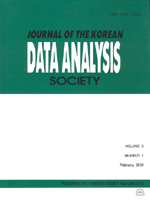상세검색
최근 검색어 전체 삭제
다국어입력
즐겨찾기0KCI등재 학술저널

# Generalized Partially Linear Single-index Model for Analysis of the Prevalence of Korean Type 2 Diabetes

• 등재여부 : KCI등재
• 2016.02
• 1 - 8 (8 pages)

The relationship between the response and the risk factors in regression model may be very complex in real applications and linear terms may not be enough to capture the relationship. In this paper we allow both a linear term and a nonlinear term of single-index in the model. Then we propose a semiparametric estimation for the generalized partially linear single-index model for binary response data. We use an efficient computation based on partial spline method in a lower-dimensional approximating function space to estimate a unknown function and regression parameters. The project pursuit regression method is used to estimate single-index parameters. The proposed method is investigated through simulation studies and the real analysis of Korean type 2 diabetes data. In real data analysis, the estimation of single-index link function showed a nonlinear trend with some curvature. It indicates the necessity of a nonlinear term of single-index. This method can be useful in a preliminary analysis to determine whether there is a nonlinear trend of single-index with risk factors in a logistic-type study.

1. Introduction

2. Estimation

3. Computation

4. Simulation

5. Data Analysis

6. Conclusion

References